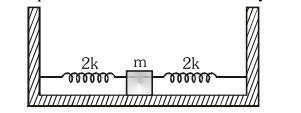# Two identical springs of spring constant '`
Question:

Two identical springs of spring constant ' $2 \mathrm{k}$ ' are attached to a block of mass $m$ and to fixed support (see figure).When the mass is displaced from equilibrium position on either side, it executes simple harmonic motion. The time period of oscillations of this sytem is:1. $2 \pi \sqrt{\frac{m}{k}}$

2. $\pi \sqrt{\frac{m}{2 k}}$

3. $2 \pi \sqrt{\frac{m}{2 k}}$

4. $\pi \sqrt{\frac{\mathrm{m}}{\mathrm{k}}}$

Correct Option: 4,

Solution:

(4) For parallel combination $k_{e q}=k_{1}+k_{2}$

$\mathrm{k}_{\mathrm{eq}}=4 \mathrm{k}$

$\mathrm{T}=2 \pi \sqrt{\frac{\mathrm{m}}{\mathrm{k}_{\mathrm{eq}}}}$